### IMO Shortlist 2017 problem A3

Kvaliteta:
Avg: 0.0
Težina:
Avg: 7.0

Let$S$ be a finite set, and let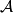$\mathcal{A}$ be the set of all functions from$S$ to$S$. Let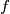$f$ be an element of$\mathcal{A}$, and let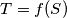$T=f(S)$ be the image of$S$ under$f$. Suppose that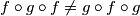$f\circ g\circ f\ne g\circ f\circ g$ for every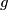$g$ in$\mathcal{A}$ with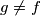$g\ne f$. Show that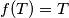$f(T)=T$.

Source: https://www.imo-official.org/problems/IMO2017SL.pdf